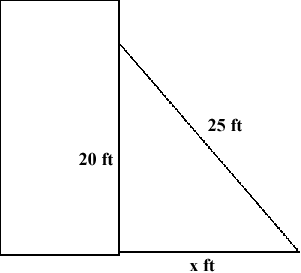SEARCH HOMEMath Central Quandaries & QueriesQuestion from Tanner, a student: a 25 foot ladder reaches a window 20 feet high. how far is the ladder from the building? how far must the foot of the ladder be moved to lower the top of the ladder by four feet?Hello Tanner,
This question has some hidden assumptions:

1. The building is built perfectly vertical (perpendicular to the ground)
2. The ground next to the building is level

A picture of our problem would be handy.So, since the building is perfectly vertical and the ground is level, this is a right angle triangle so we may use the Pythagorean Theorem to solve for x:

x2 + 202 = 252

If the ladder is moved down by 4 feet, how high is the top of the ladder from the ground?
Solve for the new x value similarly and find the difference between the two.

TylerMath Central is supported by the University of Regina and The Pacific Institute for the Mathematical Sciences.